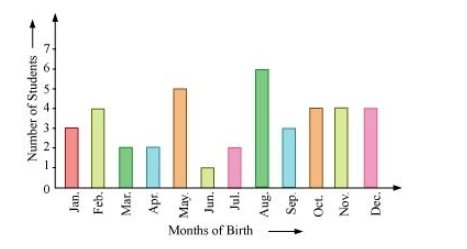# In a particular section of Class IX,

Question.

In a particular section of Class IX, 40 students were asked about the months of their birth and the following graph was prepared for the data so obtained:Find the probability that a student of the class was born in August.

Solution:

Number of students born in the month of August = 6

Total number of students = 40

P $($ Students born in the month of August $)=\frac{\text { Number of students born in August }}{\text { Total number of students }}$

$=\frac{6}{40}=\frac{3}{20}$